ICSE Class 9 Maths Sample Question Paper 6 with Answers

Section – A [40-Marks]
(Attempt all questions from this Section)

Question 1.
(a) Prove that log (1 + 2 + 3) = log 1 + log 2 + log 3.
log (1 + 2 + 3) = log 6 = log (1 x 2 x 3)
= log 1 + log 2 + log 3.(b) In the given figure, CD is a diameter which meets the chord AB in E such that
AE = BE = 4 cm. If CE = 3 cm, find the radius of the circle.Given : AE = BE = 4 cm, CE = 3 cm
Let r be the radius (OB = OC)
OE = OC – CE = r – 3.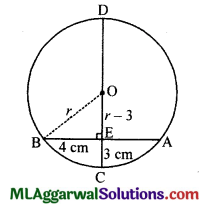⇒ OB2 = OE2 + BE2 (Pythagoras theorem)
⇒ r2 = (r – 3)2 + 42 r2
⇒ r2 – 6r + 9 + 16
⇒ 6r = 25
$$r=\frac{25}{6}=4 \frac{1}{6} \mathrm{~cm}$$(c) If ₹ 6,400 is invested at 6 $$\frac{1}{4}$$ % p.a. compound interest, find (i) the amount after 2 years (ii) the interest earned in 2 years.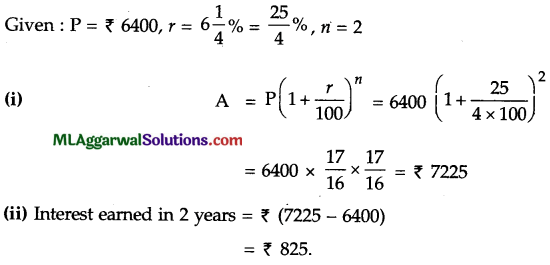Question 2.
(a) Evaluate tan x and cos y from the given figure.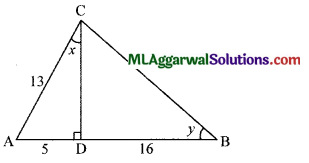In ΔACD, AC2 = AD2 + CD2
132 – 52 + CD2
⇒ CD2 = 169 – 25 = 144
⇒ CD = 12.
In A BCD, BC2 = CD2 + BD2
= 144 + 162 = 144 + 256 = 400
BC =20(b) ABC is an isosceles triangle in which altitudes BE and CF are drawn to equal sides AC and AB respectively. Show that the altitudes are equal.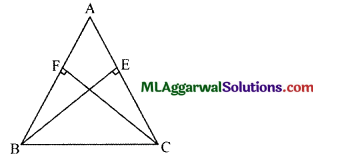Given: AC=AB
∴ In ΔBEC and ΔCFB,
∠C=∠B (∵ AB = AC)
∠BEC = ∠CFB (Each being a right angle)
BC = BC (Common side)
∴ ΔBFC  ≅ ΔCFB (AAS axiom)
∴ BE = CF (c.p.ct.)
Hence Proved.(c) The mean of 5 observations is 15. If the mean of first three observations is 14 and that of the last three is 17, find the third observation.
Mean of 5 observations = 15
∴ Sum of 5 observations = 15 x 5 = 75
Mean of first 3 observations = 14
∴ Sum of first 3 observations = 14 x 3 = 42
Mean of last 3 observations = 17
∴ Sum of last 3 observations = 17 x 3 = 51
∴ The third observation = (42 + 51) – 75 = 18.

Question 3.
(a) Factorize : x4 + 4
x4 + 4 = (x4 + 4x2 + 4) – 4x2 = {(x2)2 + 2 .x. 2 + (2)2} – (2x)2
= (x2 + 2)2 – (2x)2 = (x2 + 2 + 2x) (x2 + 2 – 2x)
= (x2 + 2x+ 2) (x2 – 2x+ 2)(b) Evaluate : $$\frac{\sin 30^{\circ}-\sin 90^{\circ}+2 \cos 0^{\circ}}{\tan 30^{\circ} \cdot \tan 60^{\circ}}$$(c) Simplify:$$(81)^{3 / 4}-3 \times(7)^{0}-\left(\frac{1}{27}\right)^{-2 / 3}$$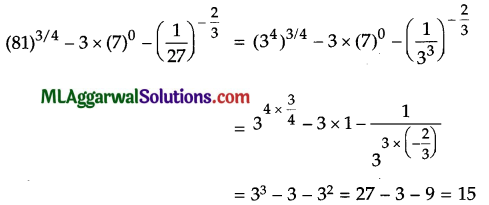Question 4.
(a) If x $$\frac{2}{x}$$ = 5, find the value of $$x^{3}-\frac{8}{x^{3}}$$(b) If the hypotenuse of a right angled triangle is 6 m more than twice the shortest side and third side is 2 m less than hypotenuse, find the sides of the triangle.
Let the shortest side be x m.
Then, Hypotenuse =(2x+6)cm,thirdside=2x+6-2=(2x+4)m.
∴ (2x + 6)2 = (2x + 4)2 + x2 (Using Pythagoras theorem)
= (2x)2 + 2.2x.6 + 62 = (2x)2 + 2.2xA +42 + x2
= 4x2 + 24x + 36 = 4x2 + 16x + 16 + x2
= 24x – 16x = x2 + 16 – 36
= x2 – 8x – 20 = 0
= x2 – (10 – 2) x – 20 =0
= x2 – 10x + 2x – 20 =0
= x (x – 10) + 2 (x – 10) = 0
= (x – 10) (x + 2) = 0
= x – 10 =0 or x + 2 = 0
= x = 10 or x = – 2
∴ x = 10 (∵ x cannot be negative)
∴ 2x + 6 = 2 x 10 + 6 = 26
and 2x + 4 = 2 x 10 + 4 = 24
Therefore, the sides are 10 m, 26 m and 24 m.(c) Simplify: $$\frac{3}{\sqrt{6}+\sqrt{3}}-\frac{4}{\sqrt{6}+\sqrt{2}}+\frac{1}{\sqrt{3}+\sqrt{2}}$$Section – B
(Attempt any four questions from this Section)

Question 5.
(a) Solve : log10 6 + log10 (4x + 5) = log10 (2x + 7) +1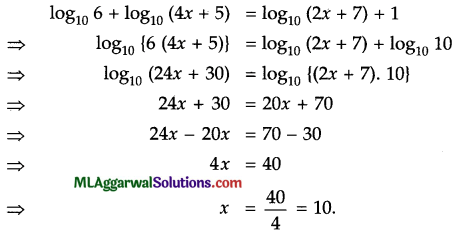(b) 3 men and 4 women can do a piece of work in 14 days while 4 men and 6 women can do it in 10 days. How long would it take 1 woman to finish the work ?
Let 1 man take x days and 1 woman take y days to finish the work.
∴ In 1 day, 1 man does = $$\frac{1}{x}$$ work and 1 woman does = $$\frac{1}{y}$$ work.
So, 3 men and 4 women do=$$3 \times \frac{1}{x}+4 \times \frac{1}{y}=\frac{3}{x}+\frac{4}{y}$$
It is given that 3 men and 4 women finish the work in 14 days.
$$\frac{3}{x}+\frac{4}{y}=\frac{1}{14}$$ ………….(i)
Also, 4 men and 6 women do the work in 10 days.
= $$\frac{4}{x}+\frac{6}{y}=\frac{1}{10}$$ …………(ii)
Multiplying equation (i) by 4 and equation (ii) by 3, we get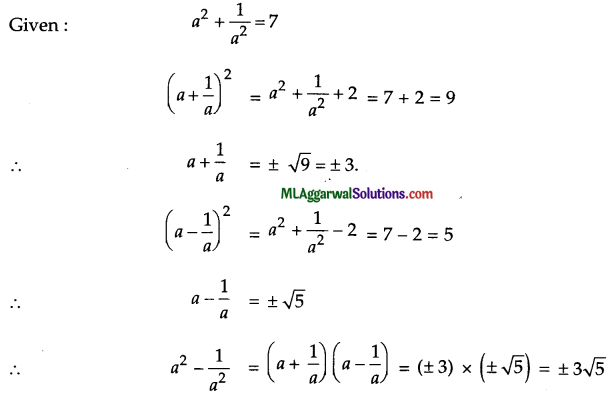∴ One woman finish the work in 140 days.(c) There are two regular polygons with number of sides equal to (n – 1) and (n + 2). Their exterior angles differ by 6°. Find the value of n
For first polygon,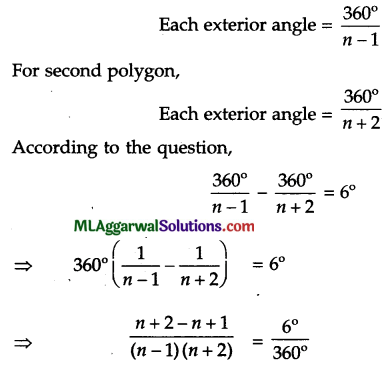$$\frac{3}{n^{2}+2 n-n-2}=\frac{1}{60}$$
n2  + n – 2 = 180
n2 + n- 182 = 0
n2  + (14 – 13) n – 182 = 0
n2  + 14n – 13n – 182 = 0
n(n + 14) -13 (n + 14) = 0
(n + 14) (n – 13) = 0
n + 14 = 0 or n – 13 =0
n = -14 = 0  or n = 13 (∵n cannot be negative)
∴ n = 13.Question 6.
(a) If $$a^{2}+\frac{1}{a^{2}}=7$$, find the value of $$a^{2}-\frac{1}{a^{2}}$$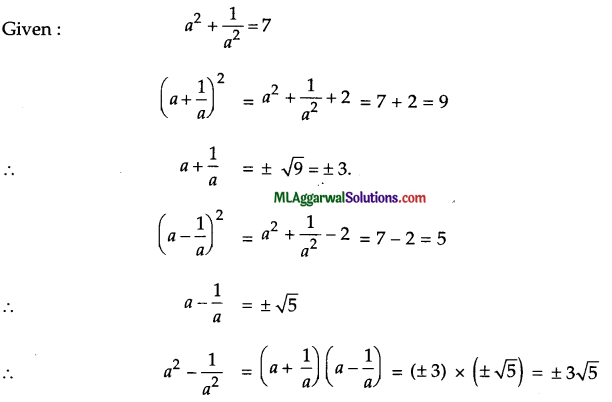(b) Construct a trapezium ABCD in which AD || BC, Z B = 60°, AB = 5 cm, BC = 6.2 cm, and CD = 4.8 cm.
Given : AD||BC, ZB = 60°, AB = 5 cm, BC = 6.2 cm, and CD = 4.8 cm.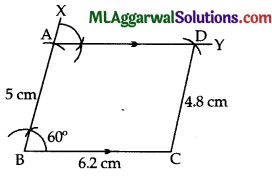Steps of construction :
(1) Draw BC = 6.2 cm.
(2) At B, draw ∠CBX = 60° and cut off BA = 5 cm.
(3) At A, draw exterior ∠XAY = 60° such that AY||BC.
(4) From C, cut-off AY at D such that CD = 4.8 cm and join CD.
Hence, ABCD is the required trapezium.(c) The inner dimensions of a closed wooden box are 2 m, 1.2 m and 0.75 m. The thickness of the wood is 2.5 cm. Find the cost of wood required to make the box if 1m3 of wood costs ₹ 5400.
The inner dimensions of the closed box are 2 m, 1.2 m, 0.75 m.
Inner volume = (2 x 1.2 x 0.75) m3 = 1.8 m3
Thickness of the box = 2.5 cm = 2.5/100m= 0.025 m
∴ Outer dimensions are (2 + 2 x 0.025) m, (1.2 + 2 x 0.025) m, (0.75 + 2 x 0.025) m
i.e. 2.05 m, 1.25, 0.8 m.
∴ Outer volume = (2.05 x 1.25 x 0.8) m3 = 2.05 m3
Volume of wood = (2.05 – 1.8) m3 0.25 m3
Cost of 1 m3 of wood = ₹ 5400
Cost of 0.25 m3 of wood = ₹5400 x 0.25
= ₹ 1350

Question 7.
(a) Solve : 4x2 + 15 =16x
4x2+15 =16x
4x2 – 16x+15 =0
4x2 – (10-t-6)x+15 =0
4x2 10x – 6x+15 =0
= 2x(2x – 5)- 3(2x – 5) =0
(2x – 5)(2x – 3) =0
2x – 5 =0 or 2x – 3=0
2x =5 or 2x=3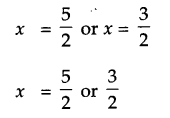(b) Find graphically the vertices of the triangle whose sides have equations
2y – x = 8, 5y – x = 14 and y – 2x = 1.
Given equations are,
2y – x =8 ……….(i)
5y – x =14 …(ii)
and y – 2x =1 …(iii)
From(i), x =2y – 8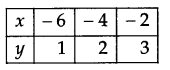∴ (- 6, 1), (- 4, 2), (- 2, 3)
From (ii), x=2 y-8(- 4, 2), (1, 3), (6, 4)
From (iii) y=2 x+1∴ (1, 3), (2, 5), (- 1, – 1)
These points are plotted on the graph.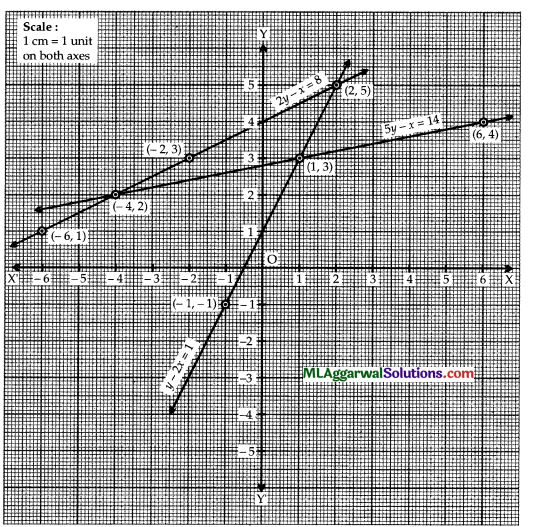The three lines intersect at point (- 4, 2), (1, 3) and (2, 5) which are the required vertices of triangle formed by them(c) If 3tan2 θ-1=0′, find cos 2θ, given that θ is acute.
Given:  3tan2 θ-1=0
tan2θ = 1/3
tan θ   =$$\frac{1}{\sqrt{3}}$$]
⇒ tanθ = tan 30°
θ = 30°
cos2θ = cos (2 x 30°) = cos 60° =$$\frac{1}{2}$$

Question 8.
(a) Solve for x : 3(2x + 1) – 2x+2 + 5 = 0.
⇒ 3(2x + 1) – 2x + 2 + 5 =0
⇒ 3.2x + 3 – 2x. 22 + 5 =0
⇒ 3.2x – 4.2x + 8=0
⇒ -2x = – 8
⇒ 2x = 23
⇒ x =3

(b) Find the area of a triangle whose perimeter is 22 cm, one side is 9 cm and the difference of the other two sides is 3 cm.
One side = 9 cm, perimeter = 22 cm.
Let other two sides be a cm and b cm and a > b.
According to the question,
a + b + 9 = 22
⇒ a + b = 13 ………..(i)
and a – b =3 (Given) ………(ii)
Adding equations (i) and (ii), we have
2 a = 16 ⇒ a = 8
Subtracting equation (ii) from equation (i), we have
2b = 10 ⇒ b = 5
The sides are a = 8 cm, b = 5 cm, c = 9 cm(c) Insert four irrational numbers between 2√3 and 3√2Question 9.
(a) Form a cumulative frequency distribution table from the following data by exclusive method taking 4 as the magnitude of class intervals.
31, 23, 19, 29, 20, 16, 10, 13, 34, 38, 33, 28, 21, 15, 18, 36, 24, 18, 15, 12, 30, 27, 23, 20, 17, 14, 32, 26, 25, 18, 29, 24, 19, 16, 11, 22, 15, 17, 10, 25.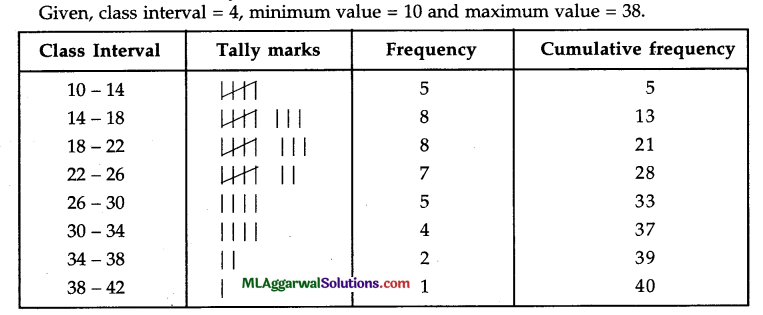(b) Solve simultaneously : $$\frac{2}{x}+\frac{2}{3 y}=\frac{1}{6} ; \frac{3}{x}+\frac{4}{y}=-\frac{1}{2}$$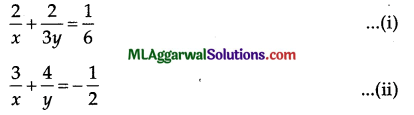(c) The diagonals AC and BD of a quadrilateral ABCD intersect at O in such a way that area of ΔOAD = area of ΔOBC. Prove that ΔBCD is a trapezium.Given: Area of ΔOAD = area of ΔOBC.
Draw DM ⊥ AB,CN ⊥AB.
∴ DM || CN (∵Both DM, CN are perpendicular to AB)
Now,
Area of ΔOAD = Area of ΔOBC
Area of ΔOAD + Area of ΔOAB = Area of ΔOBC + Area of ΔOAB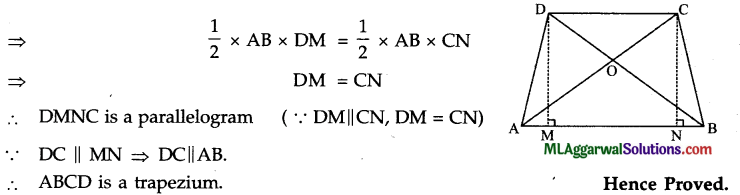Question 10.
(a) If the interest is compounded half yearly, calculate the amount when the principal is ₹ 7400, the rate of interest is 5% p. a. and the duration is one year.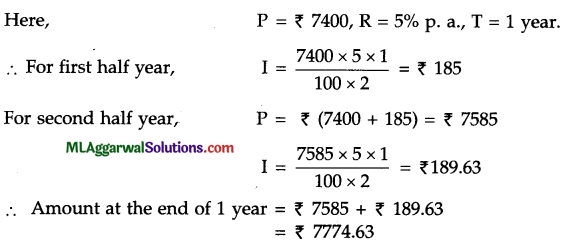(b) Find the coefficient of x2 and x in the product of (x – 2) (x – 3) (x – 4).
Given :  (x -2) (x – 3) {x – 4)
Here,  a = – 2, b = – 3, c = -4
Coefficient of x2 = a + b + c = (- 2) + (- 3) + (- 4) = – 9
Coefficient of x = ab + be + ca = (- 2) (- 3) + (- 3) (- 4) + (- 4) (- 2)
= 6 + 12 + 8 = 26

(c) If the figure given, ABCD is a trapezium in which AB || DC. P is the mid-point of AD and PR || AB. Prove that PR = $$\frac{1}{2} (AB + CD)$$.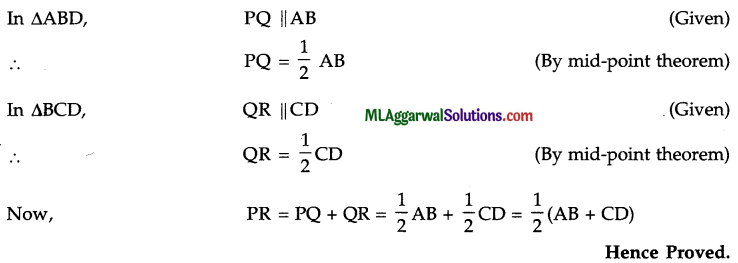Question 11.
(a) Factorize : a3 + 3a2b + 3ab2 + 2b3.
a3 + 3a2b + 3 ab2 + 2b3 = (a3 + 3 a2b + 3ab2 + b3) + b3
= (a + b)3 + (b)3 = (a + b + b) {(a + b)2 – (a + b)b + b2}
= (a + 2b) (a2 + 2ab + b2 – ab – b2 + b2)
= (a + 2b) {a2 + ab + b2)(b) In the point A (2, – 4) is equidistant from the points P (3, 8) and Q (- 10, y), find the values of y.
Given points are A (2, – 4), P (3, 8), Q (- 10, y)
AQ = AP
⇒ AQ2 = AP2
(- 10 – 2)2 + (y + 4)2
= (3 – 2)2 + (8 + 4)2 144 + (y + 4)2
= 1 + 144 (y + 4)2 = 1 y + 4 = ±1
y + 4= 1 or y + 4 = -1 y = – 3 or y = – 5
y = – 3 or – 5

(c) Simplify: $$\sqrt[a b]{\frac{x^{a}}{x^{b}}} \cdot b \sqrt[x]{\frac{x^{b}}{x^{c}}} \cdot \sqrt[c a]{\frac{x^{c}}{x^{a}}}$$